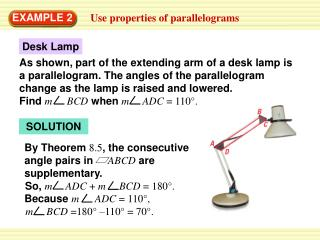Download PresentationEXAMPLE 2

# EXAMPLE 2 - PowerPoint PPT PresentationDownload Presentation## EXAMPLE 2

- - - - - - - - - - - - - - - - - - - - - - - - - - - E N D - - - - - - - - - - - - - - - - - - - - - - - - - - -
##### Presentation Transcript

1. Desk Lamp As shown, part of the extending arm of a desk lamp is a parallelogram. The angles of the parallelogram change as the lamp is raised and lowered. Find m BCDwhen m ADC = 110°. By Theorem 8.5, the consecutive angle pairs in ABCD are supplementary. So, m ADC + m BCD = 180°. Because m ADC = 110°, m BCD =180° –110° = 70°. EXAMPLE 2 Use properties of parallelograms SOLUTION

2. By Theorem 8 .6, the diagonals of a parallelogram bisect each other. So, Pis the midpoint of diagonals LNand OM. Use the Midpoint Formula. 4 + 0 7 7 + 0 , , 2 Coordinates of midpoint Pof OM = ( ) ( ) = 2 2 2 ANSWER The correct answer is A. Standardized Test Practice EXAMPLE 3 SOLUTION

3. Find the indicated measure in JKLM. 6. 3. 4. 5. NM m KML KM m JML 4 70° 40° 2 ANSWER ANSWER ANSWER ANSWER for Examples 2 and 3 GUIDED PRACTICE